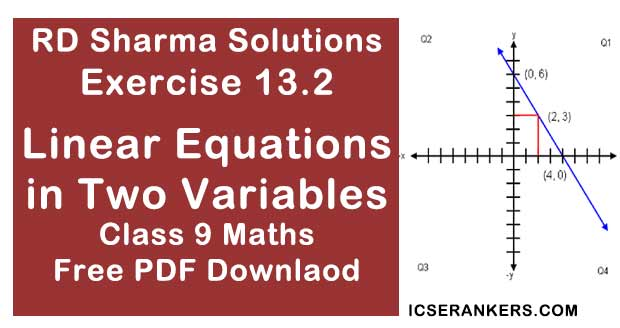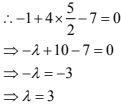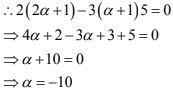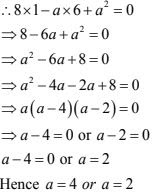# Chapter 13 Linear Equations in Two Variables RD Sharma Solutions Exercise 13.2 Class 9 MathsChapter Name RD Sharma Chapter 13 Linear Equations in Two Variables Exercise 13.2 Book Name RD Sharma Mathematics for Class 10 Other Exercises Exercise 13.1Exercise 13.3Exercise 13.4 Related Study NCERT Solutions for Class 10 Maths

### Exercise 13.2 Solutions

1. Write two solutions for each of the following equations:
(i) 3x + 4y = 7
(ii) x = 6y
(iii) x + πy = 4
(iv) 2x/3 - y = 4
Solution
(i) Given that 3x + 4y = 7
Substituting x = 0 in this equation, we get
3 × 0 + 4y = 7
⇒ y = 7/4
So, (0, 7/4) is a solution of the given equation substituting x = 1, in given equation, we
get
⇒ 3 ×1 + 4y = 7
⇒ 4y = 7 - 3 = 4
⇒ y = 1
So, (1, 1) is a solution of the given equation
∴ (0, 7/4) and (1, 1) are the solutions for the given equation

(ii) We have
x = 6y
Substituting y = 0 in this equation, we get x = 6 × 0 = 0
So, (0, 0) is a function of the given equation substituting y = 1, in the given equation, we
set x = 6 × 1 = 6
So, (6, 1) is a solution of the given equation.
∴ we obtain (0, 0) and (6, 1) as solutions of the given equation.

(iii) We have
x +  πy = 4
Substituting y = 0 in this equation, we get
x + π(0) = 4
⇒ x = 4
So, (y, 0) is a solution of the give equation.
∴ We obtain (4, 0) and (4, -x) as solutions of the given equation.

(iv) Given that
2x/3 - y = 4
Substituting y = 0 in this equation we get
2x/3 - 0 = 4
⇒ x = 4×3/2
⇒ x = 6
So, (6, 0) is a solution of the given equation
Substituting y = 1 in the given equation, we get
2/3 × -1 = 4
2x/3 = 5
⇒ x = 15/2
So, (15/2, 1) is a solution of the given equation.
∴ We obtain (6, 0) and (15/2, 1) as solutions of the given equation.

2. Write two solutions of the form x =0, y = a and x = b, y = 0 for each of the following equations:
(i) 5x - 2y = 10
(ii) -4x + 3y = 12
(iii) 2x + 3y = 24
Solution
(i) Given that
5x - 2y = 10
Substituting x = 0 in the equation 5x - 2y = 10
We get 5 ×0 - 2y = 10
⇒ y = -10/2 = -5
Thus x = 0 and y = -5 is a solution of 5x - 2y = 10
Substituting y = 0, we get
⇒ 5x - 2 × 0 = 10
⇒ 5x = 10
⇒ x = 2
Thus, x = 2 and y = 0 is a solution of 5x - 2y = 10
Thus x = 0, y = -5 and x = 2, y = 0 are two solutions of 5x - 2y = 10

(ii) Given that ,
-4x + 3y = 12
Substituting x = 0  in the equation
-4x + 3y = 12, we get
⇒ -4 × 0 + 3y = 12
⇒ 3y = 12
⇒ y = 4
Thus x = 0 and y = 4 is a solution of -4x + 3y = 12
Substituting y = 0 in the equation
-4x + 3y = 12, we get
⇒-4x + 3× 0 = 12
⇒ -4x = 12
⇒ x = 12/-4 = - 3
Thus, x = -3 and y = 0 is a solution of -4x + 3y = 12.
Thus x = 0, y = 4 and x = -3, y = 0 are two solutions of -4x + 3y = 12

(iii) Given that
2x +3y = 24
Substituting x = 0 in the given equation
2x + 3y = 24, We get
⇒ 2 × 0 + 3y = 24
⇒ 3y = 24
⇒ y = 24/3 = 8
Thus, x = 0 and y = 8 is a solution of 2x + 3y = 24
Substituting y = 0 in 2x + 3y = 24, we get 2x + 3 × 0 = 24
⇒ 2x = 24
⇒ x = 24/2 = 12
Thus x = 12 and y= 0 is a solution of 2x + 3y = 24
Thus x = 0, y = -8 and x = 12, y = 0 are two solutions of 2x + 3y = 24

3. Check which of the following are solutions of the equation 2x – y = 6 and which are not:
(i) (3, 0)
(ii) (0, 6)
(iii) (2, -2
(iv) (√3, 0)
Solution
In the equation 2x - y = 6 we get
LHS = 2x - y and RHS = 6
(i) Substituting x = 3 and y = 0 in 2x - y = 6, we get
LHS = 2 × 3 - 0 = 6 - 0 = 6 = RHS
So, x = 3, y = 0 or (3, 0) is a solution of 2x - y = 6

(ii) Substituting x = 0 and  y = 6 in 2x - y = 6, we get
LHS = 2 × 0 - 6 = -6 ≠ RHS
So, (0, 6) is not a solution of the equation 2x - y = 6

(iii) Substituting x = 2, y = -2 in 2x - y = 6, we get
LHS = 2 × 2(-2) = 4 + 2 = 6 RHS

(iv) Substituting x = √3  and y = 0 in 2x - y = 6, we get
LHS = 2 × √3 - 0 = 2√3 ≠ RHS
So, (√3, 0) is not a solution of the equation 2x - y = 6

(v) Substituting x = 1/2 and y = -5 in 2x - y = 6, we get
LHS = 2× 1/2 - (-5) = 1 + 5 = 6 = RHS
So, (1/2, -5) is a solution of the 2x - y = 6

4. If x = -1, y = 2 is a solution of the equation 3x + 4y = k, find the value of k.
Solution
Given that
3x + 4y = k
It is given that x = -1 and y = 2 is a solution of the equation 3x + 4y = k
∴ 3 × (-1) ×4 ×2 = k
⇒ -3 + 8 = k
⇒ k = 5

5. Find the value of λ, if x = -λ and y = 5/2 is a solution of the equation x + 4y - 7 = 0.
Solution
Given that
x + 4y - 7 = 0
It is given that x = -λ and y = 5/2 is a solution of the equation x + 4y - 7 = 06. If x = 2α + 1 and y = α - 1 is a solution of the equation 2x - 3y + 5 = 0, find the value of α.
Solution
We have
2x - 3y + 5 = 0
It is given that x = 2α + 1 and y = α - 1 is a solution of the equation 2x - 3y + 5 = 07. If x = 1 and y = 6 is a solution of the equation 8x - ay + a2 = 0, find the value of a.
Solution
Given that
8x - ay + a2 = 0
It is given that x = 1 and y = 6 is a solution on the equation 8x - ay + a2 = 0